Courses

# Graph Search Computer Science Engineering (CSE) Notes | EduRev

## Computer Science Engineering (CSE) : Graph Search Computer Science Engineering (CSE) Notes | EduRev

The document Graph Search Computer Science Engineering (CSE) Notes | EduRev is a part of the Computer Science Engineering (CSE) Course GATE Computer Science Engineering(CSE) 2022 Mock Test Series.
All you need of Computer Science Engineering (CSE) at this link: Computer Science Engineering (CSE)

## Graph and its representations

Graph is a data structure that consists of following two components:
1. A finite set of vertices also called as nodes.
2. A finite set of ordered pair of the form (u, v) called as edge. The pair is ordered because (u, v) is not same as (v, u) in case of directed graph(di-graph). The pair of form (u, v) indicates that there is an edge from vertex u to vertex v. The edges may contain weight/value/cost.

Graphs are used to represent many real life applications: Graphs are used to represent networks. The networks may include paths in a city or telephone network or circuit network. Graphs are also used in social networks like linkedIn, facebook. For example, in facebook, each person is represented with a vertex(or node). Each node is a structure and contains information like person id, name, gender and locale. See this for more applications of graph.

Following is an example undirected graph with 5 vertices.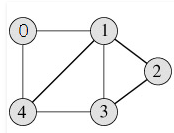Following two are the most commonly used representations of graph.
There are other representations also like, Incidence Matrix and Incidence List. The choice of the graph representation is situation specific. It totally depends on the type of operations to be performed and ease of use.

Adjacency Matrix is a 2D array of size V x V where V is the number of vertices in a graph. Let the 2D array be adj[][], a slot adj[i][j] = 1 indicates that there is an edge from vertex i to vertex j. Adjacency matrix for undirected graph is always symmetric. Adjacency Matrix is also used to represent weighted graphs. If adj[i][j] = w, then there is an edge from vertex i to vertex j with weight w.

The adjacency matrix for the above example graph is: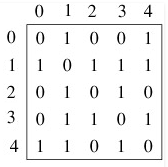Adjacency Matrix Representation of the above graph

Pros: Representation is easier to implement and follow. Removing an edge takes O(1) time. Queries like whether there is an edge from vertex ‘u’ to vertex ‘v’ are efficient and can be done O(1).

Cons: Consumes more space O(V^2). Even if the graph is sparse(contains less number of edges), it consumes the same space. Adding a vertex is O(V^2) time.

An array of linked lists is used. Size of the array is equal to number of vertices. Let the array be array[]. An entry array[i] represents the linked list of vertices adjacent to the ith vertex. This representation can also be used to represent a weighted graph. The weights of edges can be stored in nodes of linked lists. Following is adjacency list representation of the above graph.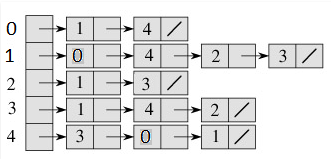Adjacency List Representation of the above Graph

Below is C code for adjacency list representation of an undirected graph:

// A C Program to demonstrate adjacency list representation of graphs

#include <stdio.h>
#include <stdlib.h>

// A structure to represent an adjacency list node
{
int dest;
};

// A structure to represent an adjacency list
{
};

// A structure to represent a graph. A graph is an array of adjacency lists.
// Size of array will be V (number of vertices in graph)
struct Graph
{
int V;
};

// A utility function to create a new adjacency list node
{
newNode->dest = dest;
newNode->next = NULL;
return newNode;
}

// A utility function that creates a graph of V vertices
struct Graph* createGraph(int V)
{
struct Graph* graph = (struct Graph*) malloc(sizeof(struct Graph));
graph->V = V;

// Create an array of adjacency lists.  Size of array will be V

// Initialize each adjacency list as empty by making head as NULL
int i;
for (i = 0; i < V; ++i)

return graph;
}

// Adds an edge to an undirected graph
void addEdge(struct Graph* graph, int src, int dest)
{
// list of src.  The node is added at the begining

// Since graph is undirected, add an edge from dest to src also
}

// A utility function to print the adjacenncy list representation of graph
void printGraph(struct Graph* graph)
{
int v;
for (v = 0; v < graph->V; ++v)
{
while (pCrawl)
{
printf("-> %d", pCrawl->dest);
pCrawl = pCrawl->next;
}
printf(" ");
}
}

// Driver program to test above functions
int main()
{
// create the graph given in above fugure
int V = 5;
struct Graph* graph = createGraph(V);

// print the adjacency list representation of the above graph
printGraph(graph);

return 0;
}

Output:

head -> 4-> 3-> 2-> 0

Pros: Saves space O(|V|+|E|) . In the worst case, there can be C(V, 2) number of edges in a graph thus consuming O(V^2) space. Adding a vertex is easier.

Cons: Queries like whether there is an edge from vertex u to vertex v are not efficient and can be done O(V).

## Breadth First Traversal or BFS for a Graph

Breadth First Traversal (or Search) for a graph is similar to Breadth First Traversal of a tree. The only catch here is, unlike trees, graphs may contain cycles, so we may come to the same node again. To avoid processing a node more than once, we use a boolean visited array. For simplicity, it is assumed that all vertices are reachable from the starting vertex.
For example, in the following graph, we start traversal from vertex 2. When we come to vertex 0, we look for all adjacent vertices of it. 2 is also an adjacent vertex of 0. If we don’t mark visited vertices, then 2 will be processed again and it will become a non-terminating process. A Breadth First Traversal of the following graph is 2, 0, 3, 1.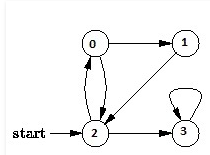Following are C++ and Java implementations of simple Breadth First Traversal from a given source.

The C++ implementation uses adjacency list representation of graphs. STL‘s list cotainer is used to store lists of adjacent nodes and queue of nodes needed for BFS traversal.

// Program to print BFS traversal from a given source vertex. BFS(int s)
// traverses vertices reachable from s.
#include<iostream>
#include <list>

using namespace std;

// This class represents a directed graph using adjacency list representation
class Graph
{
int V;    // No. of vertices
public:
Graph(int V);  // Constructor
void addEdge(int v, int w); // function to add an edge to graph
void BFS(int s);  // prints BFS traversal from a given source s
};

Graph::Graph(int V)
{
this->V = V;
}

{
}

void Graph::BFS(int s)
{
// Mark all the vertices as not visited
bool *visited = new bool[V];
for(int i = 0; i < V; i++)
visited[i] = false;

// Create a queue for BFS
list<int> queue;

// Mark the current node as visited and enqueue it
visited[s] = true;
queue.push_back(s);

// 'i' will be used to get all adjacent vertices of a vertex
list<int>::iterator i;

while(!queue.empty())
{
// Dequeue a vertex from queue and print it
s = queue.front();
cout << s << " ";
queue.pop_front();

// Get all adjacent vertices of the dequeued vertex s
// If a adjacent has not been visited, then mark it visited
// and enqueue it
{
if(!visited[*i])
{
visited[*i] = true;
queue.push_back(*i);
}
}
}
}

// Driver program to test methods of graph class
int main()
{
// Create a graph given in the above diagram
Graph g(4);

cout << "Following is Breadth First Traversal "
<< "(starting from vertex 2) ";
g.BFS(2);

return 0;
}

Output:
Following is Breadth First Traversal (starting from vertex 2)
2 0 3 1

Note that the above code traverses only the vertices reachable from a given source vertex. All the vertices may not be reachable from a given vertex (example Disconnected graph). To print all the vertices, we can modify the BFS function to do traversal starting from all nodes one by one (Like the DFS modified version).

Time Complexity: O(V+E) where V is number of vertices in the graph and E is number of edges in the graph.

## Depth First Traversal or DFS for a Graph

Depth First Traversal (or Search) for a graph is similar to Depth First Traversal of a tree. The only catch here is, unlike trees, graphs may contain cycles, so we may come to the same node again. To avoid processing a node more than once, we use a boolean visited array.
For example, in the following graph, we start traversal from vertex 2. When we come to vertex 0, we look for all adjacent vertices of it. 2 is also an adjacent vertex of 0. If we don’t mark visited vertices, then 2 will be processed again and it will become a non-terminating process. A Depth First Traversal of the following graph is 2, 0, 1, 3.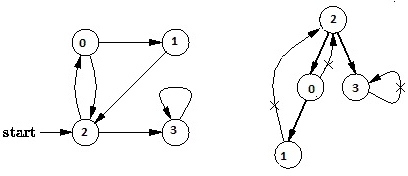Following are implementations of simple Depth First Traversal. The C++ implementation uses adjacency list representation of graphs. STL‘s listcontainer is used to store lists of adjacent nodes.

// C++ program to print DFS traversal from a given vertex in a  given graph
#include<iostream>
#include<list>

using namespace std;

// Graph class represents a directed graph using adjacency list representation
class Graph
{
int V;    // No. of vertices
void DFSUtil(int v, bool visited[]);  // A function used by DFS
public:
Graph(int V);   // Constructor
void addEdge(int v, int w);   // function to add an edge to graph
void DFS(int v);    // DFS traversal of the vertices reachable from v
};

Graph::Graph(int V)
{
this->V = V;
}

{
}

void Graph::DFSUtil(int v, bool visited[])
{
// Mark the current node as visited and print it
visited[v] = true;
cout << v << " ";

// Recur for all the vertices adjacent to this vertex
list<int>::iterator i;
if (!visited[*i])
DFSUtil(*i, visited);
}

// DFS traversal of the vertices reachable from v.
// It uses recursive DFSUtil()
void Graph::DFS(int v)
{
// Mark all the vertices as not visited
bool *visited = new bool[V];
for (int i = 0; i < V; i++)
visited[i] = false;

// Call the recursive helper function to print DFS traversal
DFSUtil(v, visited);
}

int main()
{
// Create a graph given in the above diagram
Graph g(4);

cout << "Following is Depth First Traversal (starting from vertex 2) ";
g.DFS(2);

return 0;
}

Output:
Following is Depth First Traversal (starting from vertex 2)
2 0 1 3

Note that the above code traverses only the vertices reachable from a given source vertex. All the vertices may not be reachable from a given vertex (example Disconnected graph). To do complete DFS traversal of such graphs, we must call DFSUtil() for every vertex. Also, before calling DFSUtil(), we should check if it is already printed by some other call of DFSUtil(). Following implementation does the complete graph traversal even if the nodes are unreachable. The differences from the above code are highlighted in the below code.

// C++ program to print DFS traversal for a given given graph
#include<iostream>
#include        <list>
using namespace std;

class Graph
{
int V;    // No. of vertices
void DFSUtil(int v, bool visited[]);  // A function used by DFS
public:
Graph(int V);   // Constructor
void addEdge(int v, int w);   // function to add an edge to graph
void DFS();    // prints DFS traversal of the complete graph
};

Graph::Graph(int V)
{
this->V = V;
}

{
}

void Graph::DFSUtil(int v, bool visited[])
{
// Mark the current node as visited and print it
visited[v] = true;
cout << v << " ";

// Recur for all the vertices adjacent to this vertex
list<int>::iterator i;
if(!visited[*i])
DFSUtil(*i, visited);
}

// The function to do DFS traversal. It uses recursive DFSUtil()
void Graph::DFS()
{
// Mark all the vertices as not visited
bool *visited = new bool[V];
for (int i = 0; i < V; i++)
visited[i] = false;

// Call the recursive helper function to print DFS traversal
// starting from all vertices one by one
for (int i = 0; i < V; i++)
if (visited[i] == false)
DFSUtil(i, visited);
}

int main()
{
// Create a graph given in the above diagram
Graph g(4);

cout << "Following is Depth First Traversal ";
g.DFS();

return 0;
}

Output:
Following is Depth First Traversal
0 1 2 3

Time Complexity: O(V+E) where V is number of vertices in the graph and E is number of edges in the graph.

## Applications of Depth First Search

Depth-first search (DFS) is an algorithm (or technique) for traversing a graph.

Following are the problems that use DFS as a bulding block.

1) For an unweighted graph, DFS traversal of the graph produces the minimum spanning tree and all pair shortest path tree.

2) Detecting cycle in a graph

A graph has cycle if and only if we see a back edge during DFS. So we can run DFS for the graph and check for back edges.

3) Path Finding

We can specialize the DFS algorithm to find a path between two given vertices u and z.
i) Call DFS(G, u) with u as the start vertex.
ii) Use a stack S to keep track of the path between the start vertex and the current vertex.
iii) As soon as destination vertex z is encountered, return the path as the contents of the stack

4)  Topological Sorting

Topological Sorting is mainly used for scheduling jobs from the given dependencies among jobs. In computer science, applications of this type arise in instruction scheduling, ordering of formula cell evaluation when recomputing formula values in spreadsheets, logic synthesis, determining the order of compilation tasks to perform in makefiles, data serialization, and resolving symbol dependencies in linkers .

5) To test if a graph is bipartite

We can augment either BFS or DFS when we first discover a new vertex, color it opposited its parents, and for each other edge, check it doesn’t link two vertices of the same color. The first vertex in any connected component can be red or black!

6) Finding Strongly Connected Components of a graph A directed graph is called strongly connected if there is a path from each vertex in the graph to every other vertex.

7) Solving puzzles with only one solution, such as mazes. (DFS can be adapted to find all solutions to a maze by only including nodes on the current path in the visited set.)

Offer running on EduRev: Apply code STAYHOME200 to get INR 200 off on our premium plan EduRev Infinity!

## GATE Computer Science Engineering(CSE) 2022 Mock Test Series

131 docs|159 tests

,

,

,

,

,

,

,

,

,

,

,

,

,

,

,

,

,

,

,

,

,

;